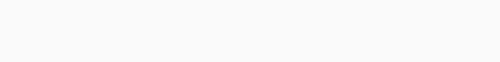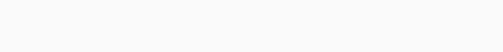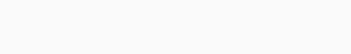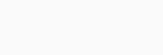# RD Sharma Class 9 Solutions Chapter 2 Exponents of Real Numbers Ex 2.1 - Learn Hool

## RD Sharma Class 9 Solutions Chapter 2 Exponents of Real Numbers Ex 2.1

These Solutions are part of RD Sharma Class 9 Solutions. Here we have given RD Sharma Class 9 Solutions Chapter 2 Exponents of Real Numbers Ex 2.1

Question 1.
Simplify the following:Solution:Question 2.
If a = 3 and b =-2, find the values of:
(i) aa+ bb
(ii) ab + ba
(iii) (a+b)ab
Solution:Question 3.
Prove that:Solution:Question 4.
Prove thatSolution:Question 5.
Prove thatSolution:Question 6.Solution:Question 7.
Simplify the following:Solution:Question 8.
Solve the following equations for x:Solution:Question 9.
Solve the following equations for x:Solution:Question 10.
If 49392 = a4b2V3, find the values.of a, b and c, where a, b and c are different positive primes.
Solution:Question 11.
If 1176 = 2a x 3b x Tc, find a, 6 and c.
Solution:Question 12.
Given 4725 = 3a5b7c, find:
(i) the integral values of a, b and c
(ii) the value of 2-a 3b 7c
Solution:Question 13.
If a = xyp-1, b = xy q-1 and c = xyr-1, prove that aq-r br-p cp-q = 1
Solution:Hope given RD Sharma Class 9 Solutions Chapter 2 Exponents of Real Numbers Ex 2.1 are helpful to complete your math homework.

If you have any doubts, please comment below. Learn Insta try to provide online math tutoring for you.

@ Designed By : Vikas Copyright 2023 @ LearnHool.In